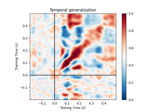# mne.decoding.PSDEstimator¶

class mne.decoding.PSDEstimator(sfreq=6.283185307179586, fmin=0, fmax=inf, bandwidth=None, adaptive=False, low_bias=True, n_jobs=1, normalization='length', verbose=None)[source]

Compute power spectral density (PSD) using a multi-taper method.

Parameters
sfreq`float`

The sampling frequency.

fmin`float`

The lower frequency of interest.

fmax`float`

The upper frequency of interest.

bandwidth`float`

The bandwidth of the multi taper windowing function in Hz.

Use adaptive weights to combine the tapered spectra into PSD (slow, use n_jobs >> 1 to speed up computation).

low_biasbool

Only use tapers with more than 90% spectral concentration within bandwidth.

n_jobs`int`

Number of parallel jobs to use (only used if adaptive=True).

normalization`str`

Either “full” or “length” (default). If “full”, the PSD will be normalized by the sampling rate as well as the length of the signal (as in nitime).

verbose

If not None, override default verbose level (see `mne.verbose()` and Logging documentation for more). If used, it should be passed as a keyword-argument only.

Methods

 `__hash__`(/) Return hash(self). `fit`(epochs_data, y) Compute power spectral density (PSD) using a multi-taper method. `fit_transform`(X[, y]) Fit to data, then transform it. `transform`(epochs_data) Compute power spectral density (PSD) using a multi-taper method.
fit(epochs_data, y)[source]

Compute power spectral density (PSD) using a multi-taper method.

Parameters
epochs_data`array`, shape (n_epochs, n_channels, n_times)

The data.

y`array`, shape (n_epochs,)

The label for each epoch.

Returns
selfinstance of `PSDEstimator`

The modified instance.

fit_transform(X, y=None, **fit_params)[source]

Fit to data, then transform it.

Fits transformer to X and y with optional parameters fit_params and returns a transformed version of X.

Parameters
X`array`, shape (n_samples, n_features)

Training set.

y`array`, shape (n_samples,)

Target values.

**fit_params`dict`

Additional fitting parameters passed to `self.fit`.

Returns
X_new`array`, shape (n_samples, n_features_new)

Transformed array.

transform(epochs_data)[source]

Compute power spectral density (PSD) using a multi-taper method.

Parameters
epochs_data`array`, shape (n_epochs, n_channels, n_times)

The data.

Returns
psd`array`, shape (n_signals, n_freqs) or (n_freqs,)

The computed PSD.

## Examples using `mne.decoding.PSDEstimator`¶